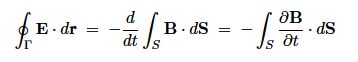# Explain Electromagnetic Induction and Faraday’s law

English physicist and chemist Michael Faraday (1791-1867) discovered that the emf produced was proportional to the rate of change of magnetic flux through the circuit. For the circuit the magnetic flux through the circuit is

ΦB = ʃS B • dS = Byx

As the loop moves, the flux decreases:

d ΦB / dt = By (dx/Dt) = – Byv

Apart from the sign (because x is decreasing), this is just the emf we found before,

Ɛ = – (d ΦB / dt)

Faraday conducted experiments of the type, as well as experiments keeping the circuit fixed and moving the magnet instead of the circuit, and experiments in which the circuit was fixed but the strength of the magnetic field was varied. In all races a current flowed, and the emf was described by Faraday’s law which is sometimes called the universal flux law, i.e. a changing magnetic flux induces an emf proportional to its rate of change. The minus sign is there because induced currents produce a magnetic field which tends to oppose the change in magnetic flux (Lenz’ law, after Russian physicist Heinrich Friedrich Emil Lenz 1804-1865) – nature resists change!

It remained a puzzle why this same formula of electromagnetic induction applied to the three quite different types of the experiment carried out by Faraday until Einstein developed his special theory of relativity.

We can write Faraday’s law aswhere loop Г bounds surface S. But, using Stokes’ theorem

ʃГ E. dr =  ʃS (Δ x E) . ds

we obtain Faraday’s law in differential form

Δ x E = – (ϐ B / ϐ t)

We see that a changing magnetic field produces an induced electric field which has Δ x E as above and Δ • E = 0. This is in contrast to the electrostatic field for which Δ x E = 0 and V • E = p/Ɛ0 — note that in the static case, i.e. (ϐ B / ϐ t) = 0, we have Δ x E = 0 as expected.